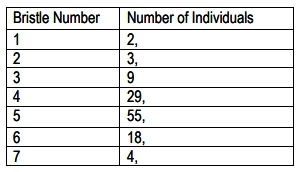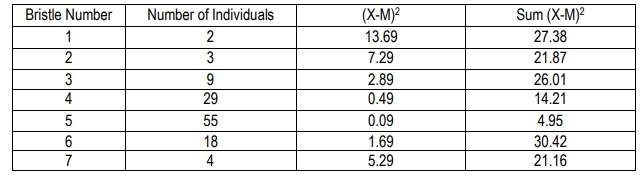Start typing, then use the up and down aroows to select an option from the list.

# Genetics

Learn the toughest concepts covered in biology with step-by-step video tutorials and practice problems by world-class tutors

20. Quantitative Genetics

# Mathematical Measurements

Next Topic1

### concept

Mathematical Measurements11m
Play a video:
0
2
Problem

The table shows a distribution of bristle numbers in a Drosophila population. What is the mean bristle number?3
Problem

The table shows a distribution of bristle numbers in a Drosophila population. What is the variance?4
Problem

Using the variance calculated in problem #2, what is the standard deviation?